NEET  >  Test: Chemical Kinetics - 1

# Test: Chemical Kinetics - 1

Test Description

## 25 Questions MCQ Test Topic-wise MCQ Tests for NEET | Test: Chemical Kinetics - 1

Test: Chemical Kinetics - 1 for NEET 2022 is part of Topic-wise MCQ Tests for NEET preparation. The Test: Chemical Kinetics - 1 questions and answers have been prepared according to the NEET exam syllabus.The Test: Chemical Kinetics - 1 MCQs are made for NEET 2022 Exam. Find important definitions, questions, notes, meanings, examples, exercises, MCQs and online tests for Test: Chemical Kinetics - 1 below.
Solutions of Test: Chemical Kinetics - 1 questions in English are available as part of our Topic-wise MCQ Tests for NEET for NEET & Test: Chemical Kinetics - 1 solutions in Hindi for Topic-wise MCQ Tests for NEET course. Download more important topics, notes, lectures and mock test series for NEET Exam by signing up for free. Attempt Test: Chemical Kinetics - 1 | 25 questions in 35 minutes | Mock test for NEET preparation | Free important questions MCQ to study Topic-wise MCQ Tests for NEET for NEET Exam | Download free PDF with solutions
 1 Crore+ students have signed up on EduRev. Have you?
Test: Chemical Kinetics - 1 - Question 1

### Which of the following statement is true for order of a reaction?

Detailed Solution for Test: Chemical Kinetics - 1 - Question 1

Order of a reaction is experimentally determined quantity so it can be negative fractional or zero.

Test: Chemical Kinetics - 1 - Question 2

### For the first order reaction, half life is equal to

Detailed Solution for Test: Chemical Kinetics - 1 - Question 2

For 1st order reaction half life is independent of initial concentration of reactant.

Test: Chemical Kinetics - 1 - Question 3

### Straight line graph for first order reaction is obtained between

Detailed Solution for Test: Chemical Kinetics - 1 - Question 3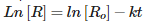Test: Chemical Kinetics - 1 - Question 4

The effect of temperature on reaction rate is given by

Detailed Solution for Test: Chemical Kinetics - 1 - Question 4

k = A exp(-kt)

Test: Chemical Kinetics - 1 - Question 5

The chemical reaction in which reactants require high amount of activation energy are generally

Detailed Solution for Test: Chemical Kinetics - 1 - Question 5

If activation energy is high rate of reaction will decrease and reaction will be slow.

Test: Chemical Kinetics - 1 - Question 6

The minimum amount of energy required by the reacting molecules at the time of collisions in order to produce effective collisions is called

Test: Chemical Kinetics - 1 - Question 7

The expression which relates the rate of reaction to the concentration of the reactants are called?

Detailed Solution for Test: Chemical Kinetics - 1 - Question 7

Rate law states that rate of reaction is dependent on concentration of reactant raise to power order of reaction.

Test: Chemical Kinetics - 1 - Question 8

The constant k used in rate equation is known as

Detailed Solution for Test: Chemical Kinetics - 1 - Question 8

Rate constant is also known as velocity constant.

Test: Chemical Kinetics - 1 - Question 9

The radioactive isotope used in determining the age of organic substances is

Detailed Solution for Test: Chemical Kinetics - 1 - Question 9

Carbon – 14 is used in determining the age of organic substances

Test: Chemical Kinetics - 1 - Question 10

The substance that slows down the reaction without being consumed is known as

Detailed Solution for Test: Chemical Kinetics - 1 - Question 10

Inhibitors inhibits the action of catalyst and hence slows down the rate of reaction.

Test: Chemical Kinetics - 1 - Question 11

Which among the following statement is not true for catalyst?

Detailed Solution for Test: Chemical Kinetics - 1 - Question 11

Catalyst doesnot change the equilibrium constant it only helps in reaching the equilibrium at a faster rate.

Test: Chemical Kinetics - 1 - Question 12

A first order reaction is 50% completed in 1.26 × 1014 s. How much time would it take for 100% completion?

Detailed Solution for Test: Chemical Kinetics - 1 - Question 12

The time taken for half the reaction to complete, i.e., the time in which the concentration of a reactant is reduced to half of its original value is called half-life period of the reaction. But it is impossible to perform 100% of the reaction. Whole of the substance never reacts because in every half-life, 50% of the substance reacts. Hence, time taken for 100% completion of a reaction is infinite.

Test: Chemical Kinetics - 1 - Question 13

Collision theory is applicable to

Detailed Solution for Test: Chemical Kinetics - 1 - Question 13

Collision theory is only applicable to bimolecular reaction.

Test: Chemical Kinetics - 1 - Question 14

Rate of reaction does not remain constant throughout because

Detailed Solution for Test: Chemical Kinetics - 1 - Question 14

Rate of reaction is dependent on concentration of reactant

Test: Chemical Kinetics - 1 - Question 15

The unit of rate constant for a first order reaction is

Detailed Solution for Test: Chemical Kinetics - 1 - Question 15

The correct answer is Option C.

Let R be the rate of reaction.
For first order reaction,
R=K[A]1
⇒K=R[A]-1
Whereas, K and [A] are rate constant and initial concentration of reactant respectively.
Therefore,
Unit of rate constant =(mol L-1)1-nsec-1
For first order reaction, n=1
Unit of rate constant = sec-1
Hence the unit of rate constant for first order reaction is sec-1.

Test: Chemical Kinetics - 1 - Question 16

Reaction which takes place in one step is known as

Detailed Solution for Test: Chemical Kinetics - 1 - Question 16

Elementary reaction are one step reactions.

Test: Chemical Kinetics - 1 - Question 17

Which among the following statement is not true for rate constant of a reaction?

Detailed Solution for Test: Chemical Kinetics - 1 - Question 17

Rate constant is independent of concentration of reactant.

Test: Chemical Kinetics - 1 - Question 18

Which among the following is an example of pseudo first order reaction?

Detailed Solution for Test: Chemical Kinetics - 1 - Question 18

In acid catalysed hydrolysis of ethylacetate the water is in excess so it is pseudo 1st order reaction.

Test: Chemical Kinetics - 1 - Question 19

Which among the following is an example of first order reaction?

Detailed Solution for Test: Chemical Kinetics - 1 - Question 19

Decomposition of N2O5 is 1st order reaction.

Test: Chemical Kinetics - 1 - Question 20

The rate law for the reaction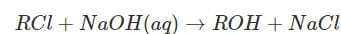is given by rate = k[RCl]. The rate for this reaction

Detailed Solution for Test: Chemical Kinetics - 1 - Question 20

Since order of reaction with respect to RCl is one so if conc of RCl is halfed the rate of reaction will also become half.

Test: Chemical Kinetics - 1 - Question 21

For an endothermic reaction, the minimum value for the energy of activation in terms of will be

Detailed Solution for Test: Chemical Kinetics - 1 - Question 21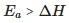Test: Chemical Kinetics - 1 - Question 22

Order of the photochemical reaction occurring between hydrogen and chlorine is

Detailed Solution for Test: Chemical Kinetics - 1 - Question 22

Photochemical reaction between H2 and Cl2 is 0 zero wrt to reactants.

Test: Chemical Kinetics - 1 - Question 23

The temperature coefficient of most of the reactions lies between

Detailed Solution for Test: Chemical Kinetics - 1 - Question 23

For every 100 rise in temperature rate of reaction increases by factor of 2 or 3.

Test: Chemical Kinetics - 1 - Question 24

Following mechanism has been proposed for a reaction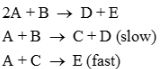The rate law expression for the reaction is

Detailed Solution for Test: Chemical Kinetics - 1 - Question 24

Rate of reaction is determined by slowest step.

Test: Chemical Kinetics - 1 - Question 25

The expression which gives 3/4th life of first order reaction is

Detailed Solution for Test: Chemical Kinetics - 1 - Question 25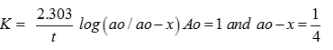## Topic-wise MCQ Tests for NEET

1493 tests
 Use Code STAYHOME200 and get INR 200 additional OFF Use Coupon Code
Information about Test: Chemical Kinetics - 1 Page
In this test you can find the Exam questions for Test: Chemical Kinetics - 1 solved & explained in the simplest way possible. Besides giving Questions and answers for Test: Chemical Kinetics - 1, EduRev gives you an ample number of Online tests for practice

## Topic-wise MCQ Tests for NEET

1493 tests

### How to Prepare for NEET

Read our guide to prepare for NEET which is created by Toppers & the best Teachers